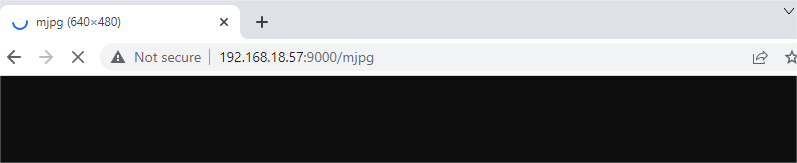# Continuous Shooting¶

This example allows us to use the pan-tilt for shooting/continuous shooting more conveniently.

By the way, a large number of still photos captured in continuous shooting can be used as frames to synthesize videos. This usage will be later in the chapter Time Lapse Photography.

Here you will use two windows at the same time: * One is Terminal, where you will enter `wasd` to control the camera orientation, enter `q` to shoot, and enter `g` to exit shooting. If the program has not been terminated after exiting the shooting, please press `ctrl+c`.

• Another is the web interface, after the program runs, you will need to enter `http://<Your Raspberry Pi IP>:9000/mjpg` in the PC browser (such as chrome) to view the viewfinder screen.

Run the Code

```cd /home/pi/pan-tilt-hat/examples
sudo python3 continuous_shooting.py
```

View the Image

After the code runs, the terminal will display the following prompt:

```No desktop !
* Environment: production
WARNING: Do not use the development server in a production environment.
Use a production WSGI server instead.
* Debug mode: off
* Running on http://0.0.0.0:9000/ (Press CTRL+C to quit)
```

Then you can enter `http://<your IP>:9000/mjpg` in the browser to view the video screen. such as: `http://192.168.18.113:9000/mjpg`Code

```#!/usr/bin/env python3
from time import sleep,strftime,localtime
from vilib import Vilib
import sys
sys.path.append('./')
from servo import Servo
import tty
import termios

fd = sys.stdin.fileno()
old_settings = termios.tcgetattr(fd)
try:
tty.setraw(sys.stdin.fileno())
finally:
return ch

manual = '''
Press keys on keyboard to record value!
W: up
A: left
S: down
D: right
Q: continuous_shooting
G: Quit
'''

# region servos init
pan = Servo(pin=13, min_angle=-90, max_angle=90) # pan_servo_pin (BCM)
tilt = Servo(pin=12, min_angle=-90, max_angle=30) # be careful to limit the angle of the steering gear
panAngle = 0
tiltAngle = 0
pan.set_angle(panAngle)
tilt.set_angle(tiltAngle)
#endregion init

# region servo control
def limit(x,min,max):
if x > max:
return max
elif x < min:
return min
else:
return x

def servo_control(key):
global panAngle,tiltAngle
if key == 'w':
tiltAngle -= 1
tiltAngle = limit(tiltAngle, -90, 30)
tilt.set_angle(tiltAngle)
if key == 's':
tiltAngle += 1
tiltAngle = limit(tiltAngle, -90, 30)
tilt.set_angle(tiltAngle)
if key == 'a':
panAngle += 1
panAngle = limit(panAngle, -90, 90)
pan.set_angle(panAngle)
if key == 'd':
panAngle -= 1
panAngle = limit(panAngle, -90, 90)
pan.set_angle(panAngle)

# endregion

# continuous shooting
def continuous_shooting(path,interval_ms:int=50,number=10):
print("continuous_shooting .. ")
path=path+'/'+strftime("%Y-%m-%d-%H.%M.%S", localtime())
for i in range(number):
Vilib.take_photo(photo_name='%03d'%i,path=path)
print("take_photo: %s"%i)
sleep(interval_ms/1000)
print("continuous_shooting done,the pictures save as %s"%path)
sleep(0.2)

def main():

Vilib.camera_start(vflip=True,hflip=True)
Vilib.display(local=True,web=True)

path = "/home/pi/Pictures/vilib/continuous_shooting"

print(manual)
while True:
servo_control(key)
if key == 'q':
continuous_shooting(path,interval_ms=50,number=10)
elif key == 'g':
Vilib.camera_close()
break
sleep(0.01)

if __name__ == "__main__":
main()
```

How it works?

The code in this article looks slightly complicated, we can split it into three parts:

• Keyboard input

• Servo control

• Take photos

1. First, let’s look at the keyboard control part, which includes the following parts:

```import sys
import tty
import termios

fd = sys.stdin.fileno()
old_settings = termios.tcgetattr(fd)
try:
tty.setraw(sys.stdin.fileno())
finally:
return ch

def main():
while True:
sleep(0.1)

if __name__ == "__main__":
main()
```

Its function is to make the terminal can obtain the keyboard input value in real time (without pressing enter), which is more convenient for practical operation.

2. Secondly, let’s look at the steering gear control part, which consists of the following code:

```from time import sleep,strftime,localtime
from servo import Servo

### The readchar part is omitted here ###

# region servos init
pan = Servo(pin=13, min_angle=-90, max_angle=90) # pan_servo_pin (BCM)
tilt = Servo(pin=12, min_angle=-90, max_angle=30) # be careful to limit the angle of the steering gear
panAngle = 0
tiltAngle = 0
pan.set_angle(panAngle)
tilt.set_angle(tiltAngle)
#endregion init

# region servo control
def limit(x,min,max):
if x > max:
return max
elif x < min:
return min
else:
return x

def servo_control(key):
global panAngle,tiltAngle
if key == 'w':
tiltAngle -= 1
tiltAngle = limit(tiltAngle, -90, 30)
tilt.set_angle(tiltAngle)
if key == 's':
tiltAngle += 1
tiltAngle = limit(tiltAngle, -90, 30)
tilt.set_angle(tiltAngle)
if key == 'a':
panAngle += 1
panAngle = limit(panAngle, -90, 90)
pan.set_angle(panAngle)
if key == 'd':
panAngle -= 1
panAngle = limit(panAngle, -90, 90)
pan.set_angle(panAngle)

# endregion

def main():
while True:
servo_control(key)

if __name__ == "__main__":
main()
```

It seems to be a little bit more complicated, but after careful observation, you will find that most of this is the initialization and restriction of the position of the steering gear, which can be perfected according to personal preferences. Its main core is nothing more than the following lines:

```from time import sleep,strftime,localtime
from servo import Servo

### The readchar part is omitted here ###

# region servos init
pan = Servo(pin=13, min_angle=-90, max_angle=90) # pan_servo_pin (BCM)
tilt = Servo(pin=12, min_angle=-90, max_angle=30) # be careful to limit the angle of the steering gear
panAngle = 0
tiltAngle = 0
pan.set_angle(panAngle)
tilt.set_angle(tiltAngle)
#endregion init
```
• And `tilt = Servo(pin=12, min_angle=-90, max_angle=30)` is used to init the servo object. Here, the servo connected to 12 is declared as an object named `tilt` .

• As for `tilt.set_angle(tiltAngle)` , it directly controls the tiltServo, which is the angle of the servo connected to 12.

3. Finally, let’s take a look at the photo section, which is roughly similar to Take Photo, but with the addition of continuous shooting.

```from time import sleep,strftime,localtime
from vilib import Vilib

### The readchar part & servo part is omitted here ###

# continuous shooting
def continuous_shooting(path,interval_ms:int=50,number=10):
print("continuous_shooting .. ")
path=path+'/'+strftime("%Y-%m-%d-%H.%M.%S", localtime())
for i in range(number):
Vilib.take_photo(photo_name='%03d'%i,path=path)
print("take_photo: %s"%i)
sleep(interval_ms/1000)
print("continuous_shooting done,the pictures save as %s"%path)
sleep(0.2)

def main():
Vilib.camera_start(vflip=True,hflip=True)
Vilib.display(local=True,web=True)

path = "/home/pi/Pictures/continuous_shooting"

while True:
#servo_control(key)
if key == 'q':
continuous_shooting(path,interval_ms=50,number=10)
if key == 'g':
Vilib.camera_close()
break
sleep(0.1)

if __name__ == "__main__":
main()
```

We have written a function `continuous_shooting(path,interval_ms=50,number=10)`, whose function is to execute a for loop and execute `Vilib.take_photo()` to achieve continuous shooting.

The photos produced by continuous shooting will be stored in a newly created folder, and the folder will be named according to the current time. Here you may be curious about the time-related functions `strftime()` and `localtime()`, then please see Time-Python Docs.